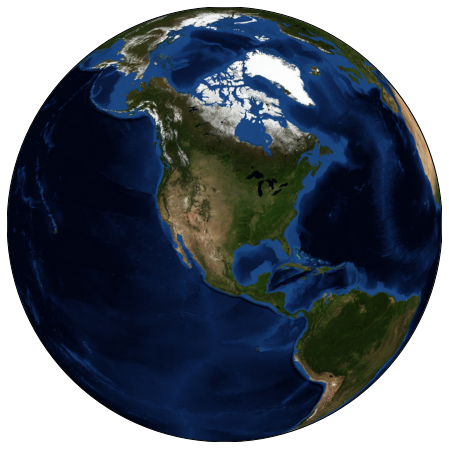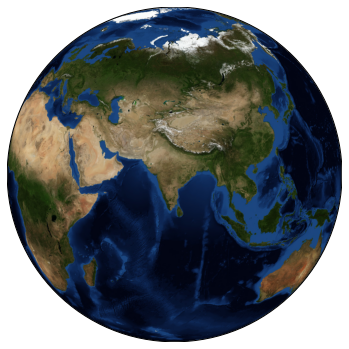# Geographical plotting with matplotlib

## Geographical plotting with `matplotlib`¶¶

To get the features, install the `basemap` package using

• `conda install basemap` and the high res dataset with
• `conda install -c conda-forge basemap-data-hires`.

I ran into multiple conflicts trying to install `basemap` in an environment with `geopandas`. I was successful in creating a new Python env and installing basemap first.

Thanks to https://jakevdp.github.io/PythonDataScienceHandbook/04.13-geographic-data-with-basemap.html for this tutorial.

In :
```%matplotlib inline
import numpy as np
import matplotlib.pyplot as plt
from mpl_toolkits.basemap import Basemap
```

### Common steps in plotting with `basemap` package¶¶

1. First you set the figure size and other properties of the matplotlib figure
2. Next you set the projection using the `Basemap` constructor. You pass the name of the projection from the choice list, a resolution of the built-in dataset to use. Higher res is slower to draw. You pass either the coordinates of lower left & upper right, or coordinates of center. This gives you a `Basemap` object which is like an axis. If you are doing a subplot, you can bind it to an existing axes.
3. On the `Basemap` object, you call the appropriate basemap type - such as `etopo()`, `bluemarble()`, `shadedrelief` etc. and pass appropriate parameters like scale, alpha etc. Scale is not map scale or tiling level, instead it is the factor to scale up or down the rendered image. The default resolution is `5400x2700` which is computational intensive. You can pass any additional `imshow` parameters to this call.
4. If you want to overlay any vectors, you call the `basemap` object like a function and pass the coordinates in lat, lon. The function will return in the appropriate projection system you used to create the object.
5. Then you use the regular `plt.plot(x,y, symbol_spec)` syntax to plot those points on the map and `plt.text(x,y, text_spec)` for labels.

#### Plot a sphere¶¶

In :
```plt.figure(figsize=(8,8))
m = Basemap(projection='ortho', resolution=None, lat_0=35, lon_0=-100)
m.bluemarble(scale=0.6);
```
```Clipping input data to the valid range for imshow with RGB data ([0..1] for floats or [0..255] for integers).
```In :
```type(m)
```
Out:
`mpl_toolkits.basemap.Basemap`

Let us set the view to India, change figure size and the resolution..

In :
```plt.figure(figsize=(6,10))
m = Basemap(projection='ortho', resolution=None, lat_0=20.59, lon_0=78.96)
m.bluemarble(scale=0.5);
```
```Clipping input data to the valid range for imshow with RGB data ([0..1] for floats or [0..255] for integers).
```In :
```type(m)
```
Out:
`mpl_toolkits.basemap.Basemap`

#### Plot and overlay annotation¶¶

Plot with a different basemap, using Lambert Conformal Conic projection

In :
```fig = plt.figure(figsize=(8, 8))
m2 = Basemap(projection='lcc', resolution=None,
width=8E6, height=8E6,
lat_0=45, lon_0=-100,)
m2.etopo(scale=0.5, alpha=0.5)

# Map (long, lat) to (x, y) for plotting
x, y = m2(-122.3, 47.6)
plt.plot(x, y, 'ok', markersize=5)
plt.text(x, y, ' Seattle', fontsize=12);
```
```Clipping input data to the valid range for imshow with RGB data ([0..1] for floats or [0..255] for integers).
```